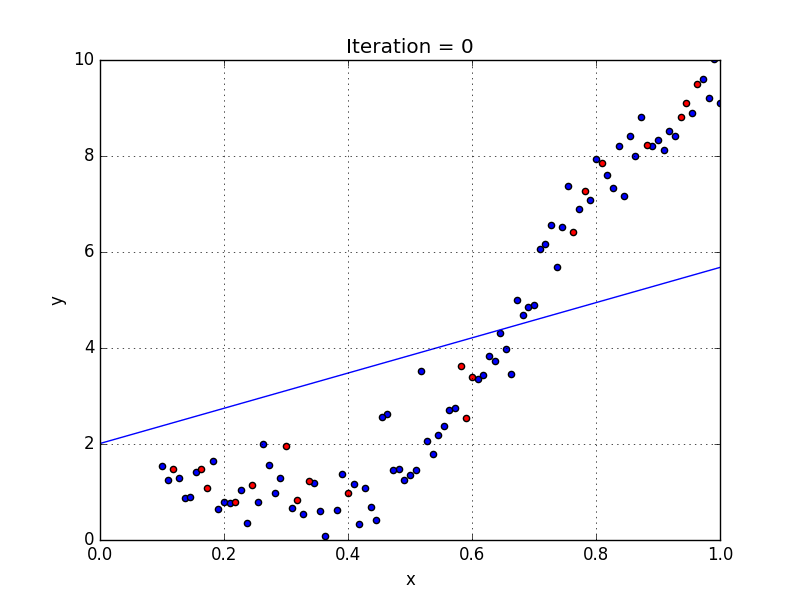# Simple Linear Regression, Cost Function & Gradient Descent

Linear regression is a powerful statistical technique and machine learning algorithm used to predict the relationship between two variables or factors usually for continuous data.Fig 1: Linear Regression | GIF: Towards Data Science

# Application of LinearRegressions

Linear Regression mainly used in understanding Business and factors influencing profitability, to evaluate trends in business and forecasts. Linear regression is used to analyze market effectiveness, pricing, and promotions of products. It is also used to assess risk in banking sectors and used in the Recommender Systems.

Let's consider a simple example of house rate prediction where a house rate is predicted based on the given area of the house.## Sanathkumar Sunkad

Research Assistant @Indian Institute of Science